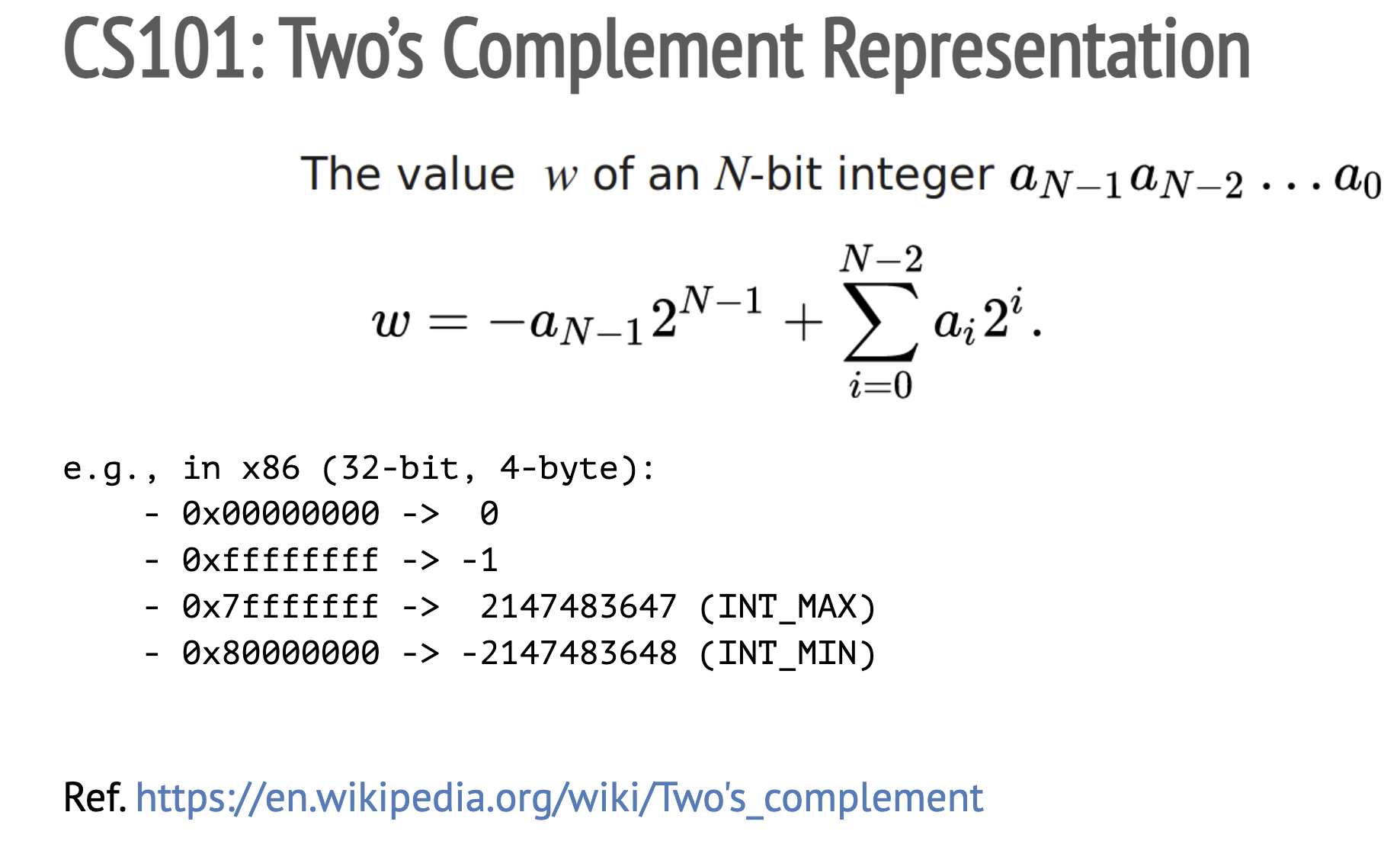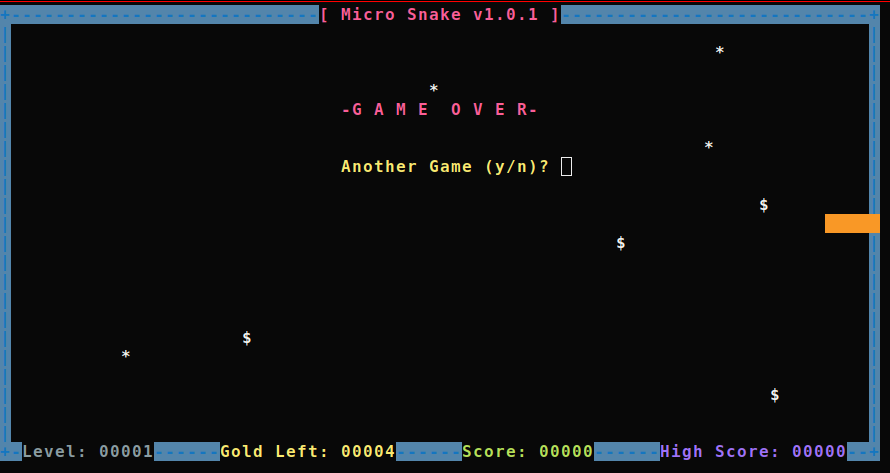# Tut08: Logic Errors

In this tutorial, we will learn about three class of popular logic bugs (i.e., non-memory safety bugs): an integer overflow, a race condition, and a command injection.

## 1. Integer overflows

Let's first take a look on `crackme0x00.c`:

``````void start() {
int passwd;
printf("IOLI Crackme Level 0x00\n");
scanf("%d", &passwd);
if (absolute(passwd) < 0) {
round2();
} else {
}
}
``````

It is asking for a password that its absolute value is less than zero: `absolute(passwd) < 0`. Note that the `absolute()` function is nothing but to convert any negative integer to its positive form by negativing the provided integer, as in the standard library:

``````// @stdlib/abs.c
/* Return the absolute value of I.  */
int absolute(int i) {
if (i<0)
return -i;
else
return i;
}
``````

Is this mathematically feasible? No. However, as our computer can only express a small part of the integer space: e.g., a 32-bit register can express 2^32 numbers of integers (more on later), this password check can be bypassed!

Let's look at the actual instructions of `absolute()`:

``````0000000000402118 <absolute>:
402118:       55                      push   rbp
402119:       48 89 e5                mov    rbp,rsp
40211c:       89 7d fc                mov    DWORD PTR [rbp-0x4],edi
40211f:       83 7d fc 00             cmp    DWORD PTR [rbp-0x4],0x0
402123:       79 07                   jns    40212c <absolute+0x14>
402125:       8b 45 fc                mov    eax,DWORD PTR [rbp-0x4]
402128:       f7 d8                   neg    eax
40212a:       eb 03                   jmp    40212f <absolute+0x17>
40212c:       8b 45 fc                mov    eax,DWORD PTR [rbp-0x4]
40212f:       5d                      pop    rbp
402130:       c3                      ret
``````

How does the machine perform the `neg eax` instruction? Unlike the arithmetic way of multiplying -1 to the multiplicand, the machine simply flips each bit (from 0 to 1 and vice versa) and adds one to the result. In two's complement representation, it happens to serve as a negation operation for most of the integers that a register can express.

For example, `0x00000001` is a positive integer `1`. If we flip every bit, it becomes `0b1111....1110`, which is `0xfffffffe` in a hexadecimal representation, and adding one to it, we get `0xffffffff`. This is the two's complement representation of `-1`.Two's complement

The biggest positive integer (i.e., `INT_MAX`) a 32-bit register can hold is `0x7fffffff`, which is 2147483647. The smallest negative integer (i.e., `INT_MIN`) is `0x80000000`, which is -2147483648 in decimal. One property that you might notice in two's complement is its asymmetry in representing the range of negative and positive integers: -2147483648 (`INT_MIN`) to 2147483647 (`INT_MAX`).

What's the arithmetic value of `-INT_MAX`? It is -2147483647. What about `-INT_MIN`? It is 2147483648, but it is bigger than `INT_MAX`! Now, let's approach this from the machine's perspective; try negating `INT_MIN` yourself by flipping the bits and adding one. Each bit in `0x80000000` are flipped, so it becomes `0x7fffffff`, and then, adding one to `0x7fffffff` results in `0x80000000`, which is `INT_MIN`. In other words, when the machine `neg`ates `INT_MIN`, it ends up returning the same `INT_MIN`! Therefore, `absolute(INT_MIN)` shown above will return `INT_MIN`, which is not a positive integer.

[Task] Phase 1 - provide a password to take the `if` branch and make the program print "Password OK :)". That will bring you to the next phase (Crackme Level 0x01).

## 2. Race condition

Once the first phase is solved, crackme0x00 goes to the next phase. In this phase, it generates a password on-the-fly (see `gen_new_passwd()` in `crackme0x00.c`) and asks for the correct password.

``````void round2() {
int passwd = gen_new_passwd();
save_passwd_into_vault(passwd);

printf("IOLI Crackme Level 0x01\n");

char buf;
scanf("%31s", buf);

if (atoi(buf) == passwd) {
printf("[!] Have a great fun!\n");
snake_main();
} else {
}
}
``````

One interesting behavior of this program is that `save_passwd_into_vault()` temporarily stores the password to `/tmp/.lock-[pid]`, and immediately removes the temporary file.

``````void save_passwd_into_vault(int passwd) {
char tmpfile;
snprintf(tmpfile, sizeof(tmpfile), "/tmp/.lock-%d", getpid());
if (access(tmpfile, F_OK) != -1) {
printf("the lock file exists, please first clean up\n");
exit(1);
}

FILE *fp = fopen(tmpfile, "w");
if (!fp)
err(1, "failed to create %s", tmpfile);
fprintf(fp, "%d", passwd);
fclose(fp);

/* DELETED! */
}
``````

How would you steal the password stored in this file? Although the lifetime of this file is very short, it is stored in a predictable location (i.e., `/tmp/.lock-[pid]`), which gives an attacker an opportunity to leak the content inside the file by having a process racing to access the same file.

PID is (likely) assigned in a sequential order so that your exploit code might bruteforce after spawning a target (with `process()` in pwntool). In fact, the parent process knows the PID of a child process even before `exec`-ing the child's process image. If you are not familiar with the concept of `fork()`, please read `man fork` before writing the exploit!

### Tip. About `template.py`

You might invoke `process()` together with `stdin=PTY` and `stdout=PTY` to manage the child process up to this stage (`man pty`). However, the `interactive()` of pwntools doesn't render the output of `snake` (next phase). We recommend using the below template for the next stage.

``````import os
import pty

(pid, fd) = pty.fork()
if pid == 0:
# child
os.execle("./target", "./target", os.environ)
exit(0)
else:
# parent
lock = "/tmp/.lock-%d" % pid

# this is how to write a message to the child
os.write(fd, "...")
``````

[Task] Phase 2 - exploit the race condition to steal the password that's generated on-the-fly. Provide the password and get "Password OK :)" printed, and get to the final phase.

## 3. Command injectionMicro Snake

Once you have passed the first two phases, you can see an Easter Egg -- an old-fashioned snake game. First, take a look at `snake/snake.c` (from Micro Snake).

Have you noticed an interesting piece of code in the `main()`?

``````// snake/snake.c
void snake_main() {
...
if (WEXITSTATUS(system ("stty cbreak -echo stop u")))
{
fprintf (stderr, "Failed setting up the screen, is 'stty' missing?\n");
return 1;
}
}
``````

Interestingly, the binary invokes a libc's `system()` when it starts (check `man stty`!). In fact, such a pattern is vulnerable to a command injection attack (e.g., in a setuid binary). How would you hijack `crackme0x00` without exploiting a memory corruption bug like previous labs?

At this point, you might realize that this technique would have come in handy when you were solving the bomb challenges (labs 1 and 2). How come?

Good luck!

[Task] Final phase - inject a command to the snake game to print the flag. Submit the flag on the submission site.